## Introduction

Prerequisites: Queue

Source on Github

A linked list is an implementation of a queue that uses a chain of pointers. Instead of storing all the data in a fixed set of memory, we can store each element by itself but have a pointer from each element to the next element. A pointer is something that holds the memory location of another object.

Imagine you were on a scavenger hunt with lots of treasure chests. You start with a clue to the first chest. Each chest contains a lot of gold (data) but it also contains a clue to the next treasure chest. To get to the fifth chest, you need to visit the first, second, third and fourth chest. This is essentially how a linked list works.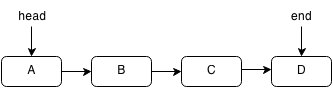A linked list is similar to an array but it is different such that it is not stored in one block of data. Each element can be stored in a random place in memory but each element contains a pointer to the next element thus forming a chain of pointers. Think of a pointer as a clue to the next chest. Since the elements aren't in a block, accessing an element must be done by traversing the entire linked list by following each pointer to the next. However, this also allows insertion and deletion to be done more quickly. In a linked list the links only go forward and you cannot move backward. However, a doubly linked list is a linked list that has pointers going backwards as well as forwards.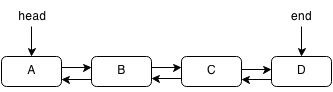Operation Get Push Delete Insert
Time Complexity O(n) O(1) O(1) O(1)

In Java, there already exists a LinkedList class but we will implement our own.

We need a Link class for each "link" in the Linked List. In each Link we only need the value and location of the previous and next node.

```class Link {
int value;

public Link(int value) {
this.value = value;
this.next = null;
}
}
```

Create the linked list by initializing the starting node and ending node as null and setting the size to empty.

```public class LinkedList {

public int size;

end = null;
size = 0;
}
}
```

## Push

Create a new node with the value given and add it to the end. We have to set the current head previous node to the new node and the new next next to the last node.

For example, if we want to insert E into the following linked list: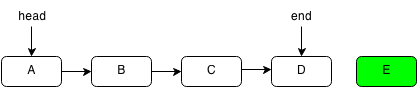First, we set the next pointer of the last element to the next element.Then we set the new end of the linked list.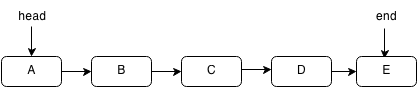```public void push(int value) {
if (size == 0) {
}
else {
}
size++;
}
```

## Pop

Pops off the head of the linked list and throws an exception if the linked list is empty.

For example, if we want to pop the first element of the following linked list:We set the head of the linked list to the second element.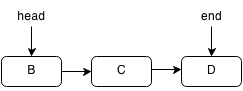```public int pop() {
if (head == null) {
throw new NoSuchElementException();
}
int ret = head.value;
size--;
if (size == 0) {
end = null;
}
return ret;
}
```

## Get

Get retrieves the value at the specified index. We have to loop through the entire list to get to the index we want.

For example, if we want to get the element C in the following linked list:

We first start at the head element and iterate through each node.We keep following the next pointers until we reach the node we want or the end of the linked list.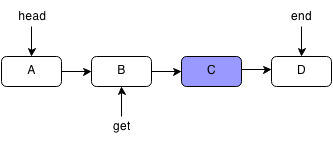Since our current pointer points to C, we stop.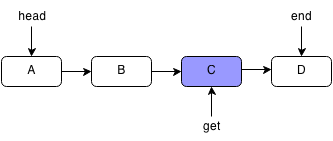```public int get(int index) {
int i = 0;
while (curNode != null) {
if (index == i) {
return curNode.value;
}
curNode = curNode.next;
i++;
}
throw new NoSuchElementException();
}
```

## Delete

To delete the current node we set the previous node next link to the link that is next.

For example, if we wanted to delete B from the following linked list: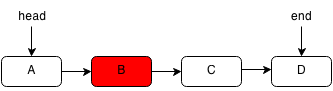We would find B and set the previous node's next to B's next which is C.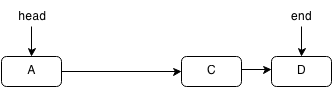```public void deleteNext(Link node) {
if (node.next == end) {
end = node;
}
node.next = node.next.next;
size--;
}
```

## Exercises

1. Implement a doubly linked list.
2. A game is played by always eliminating the kth player from the last elimination and played until one player is left. Given N players where each is assigned to a number, find the number of the last remaining player.

For example, you have 5 players (1,2,3,4,5) and the 3rd player is eliminated.

• 1, 2, 3, 4, 5
• 1, 2, 4, 5 (1, 2, 3 is eliminated)
• 2, 4, 5 (4, 5, 1 is eliminated)
• 2, 4 (2, 4, 5 is eliminated)
• 2 (2, 4, 2 is eliminated)
• Player 2 is the last one standing.
3. Given two linked lists which may share tails, determine the point at which they converge. For example, if our first linked list is [1,2,4,5] and our second linked list is [0,3,4,5] and each number is a node, then the two linked lists converge at 4.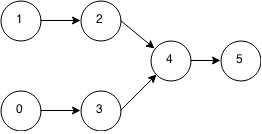4. Given a node in a doubly linked list, write a function that removes the node from the list.